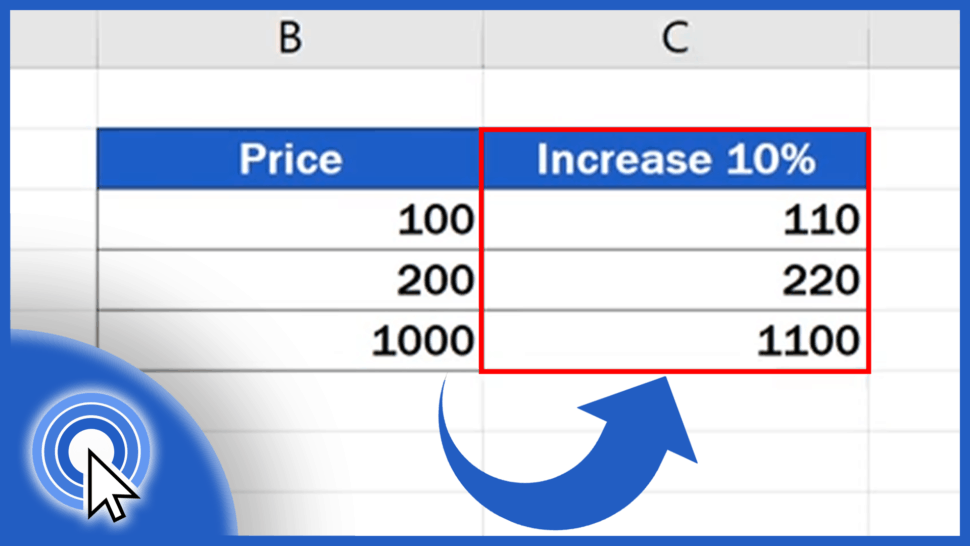# How to Calculate Percentage Increase in Excel (The Right Way)In this tutorial, we’ll learn three different ways how to calculate percentage increase in Excel. All of them are correct and can be used interchangeably to get the same result.

Let’s start!

See the video tutorial and transcription below:

Contents

Let’s say we need to increase these three values that appear in the column B by 10%, and we need the result to show in the column C.

## First Way How to Calculate Percentage Increase in Excel

One way to do this is by following these steps:

Click on the cell C3, enter the equal sign, then enter B3 multiplied by 1.1. Excel will use this formula to increase the original value in the cell B3 by 10%.

If you need to increase the value by 20%, simply multiply B3 by 1.2.

## Second Way How to Calculate Percentage Increase in Excel

And here’s the second way how to calculate percentage increase in Excel:

Go to the cell C4 and enter the equal sign again. Then click on the value you need to increase, in this case B4. We’ll multiply it by ‘110%’, which is exactly 100% as the full original price plus 10% of this value as the desired increase.

Note that this number must be stated in percent. If we want to increase the value by 30%, we’ll multiply it by ‘130%’ and that’s the trick!

Let’s move on!

## Third Way Wow to Calculate Percentage Increase in Excel

Here comes the last, third way how to calculate percentage increase in Excel.

Select the cell C5 and type in the equal sign. Carry on by entering the reference to the price we want to increase, which is B5. And now we’ll add 10% of this value by typing in the plus sign, then ‘B5’, but the value from B5 must be multiplied by 0.1.

This formula adds 10% of the value to the value itself. That’s how we can calculate the increase of a value by 10%.

And that’s it for today!

We’ve covered three different ways how to calculate percentage increase of any number.

Which of the formulas mentioned in today’s tutorial do you like best?

Share your opinion with us – leave us a comment before you go! Our team will be happy to hear from you!

Don’t miss out a great opportunity to learn:

If you found this tutorial helpful, give us a like and watch other video tutorials by EasyClick Academy. Learn how to use Excel in a quick and easy way!

Is this your first time on EasyClick? We’ll be more than happy to welcome you in our online community. Hit that Subscribe button and join the EasyClickers!

Thanks for watching and I’ll see you in the next tutorial!